# `sklearn.model_selection`.cross_val_score¶

`sklearn.model_selection.``cross_val_score`(estimator, X, y=None, groups=None, scoring=None, cv=None, n_jobs=1, verbose=0, fit_params=None, pre_dispatch='2*n_jobs')[source]

Evaluate a score by cross-validation

Read more in the User Guide.

Parameters: estimator : estimator object implementing ‘fit’ The object to use to fit the data. X : array-like The data to fit. Can be, for example a list, or an array at least 2d. y : array-like, optional, default: None The target variable to try to predict in the case of supervised learning. groups : array-like, with shape (n_samples,), optional Group labels for the samples used while splitting the dataset into train/test set. scoring : string, callable or None, optional, default: None A string (see model evaluation documentation) or a scorer callable object / function with signature `scorer(estimator, X, y)`. cv : int, cross-validation generator or an iterable, optional Determines the cross-validation splitting strategy. Possible inputs for cv are: None, to use the default 3-fold cross validation, integer, to specify the number of folds in a (Stratified)KFold, An object to be used as a cross-validation generator. An iterable yielding train, test splits. For integer/None inputs, if the estimator is a classifier and `y` is either binary or multiclass, `StratifiedKFold` is used. In all other cases, `KFold` is used. Refer User Guide for the various cross-validation strategies that can be used here. n_jobs : integer, optional The number of CPUs to use to do the computation. -1 means ‘all CPUs’. verbose : integer, optional The verbosity level. fit_params : dict, optional Parameters to pass to the fit method of the estimator. pre_dispatch : int, or string, optional Controls the number of jobs that get dispatched during parallel execution. Reducing this number can be useful to avoid an explosion of memory consumption when more jobs get dispatched than CPUs can process. This parameter can be: None, in which case all the jobs are immediately created and spawned. Use this for lightweight and fast-running jobs, to avoid delays due to on-demand spawning of the jobs An int, giving the exact number of total jobs that are spawned A string, giving an expression as a function of n_jobs, as in ‘2*n_jobs’ scores : array of float, shape=(len(list(cv)),) Array of scores of the estimator for each run of the cross validation.

`sklearn.metrics.make_scorer`
Make a scorer from a performance metric or loss function.

Examples

```>>> from sklearn import datasets, linear_model
>>> from sklearn.model_selection import cross_val_score
>>> X = diabetes.data[:150]
>>> y = diabetes.target[:150]
>>> lasso = linear_model.Lasso()
>>> print(cross_val_score(lasso, X, y))
[ 0.33150734  0.08022311  0.03531764]
```

## Examples using `sklearn.model_selection.cross_val_score`¶Imputing missing values before building an estimator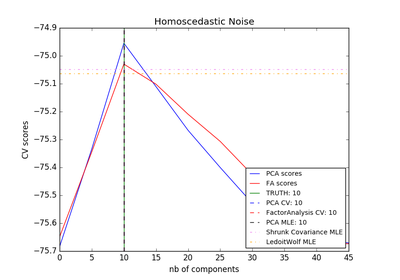Model selection with Probabilistic PCA and Factor Analysis (FA)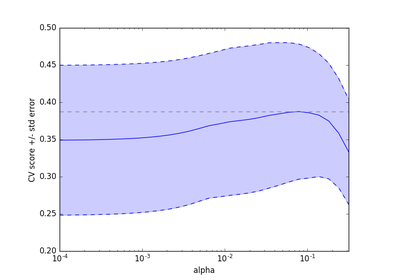Cross-validation on diabetes Dataset Exercise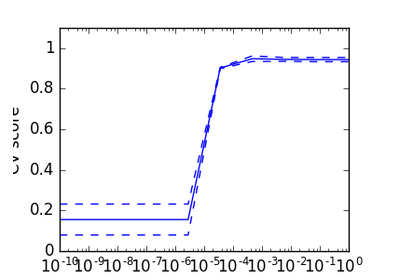Cross-validation on Digits Dataset Exercise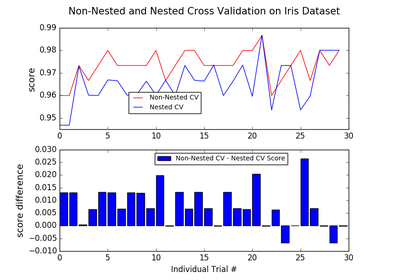Nested versus non-nested cross-validation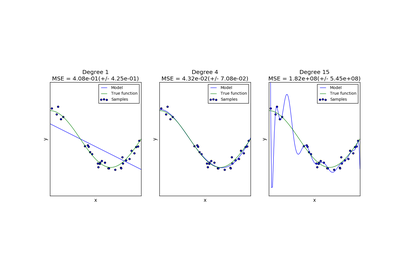Underfitting vs. Overfitting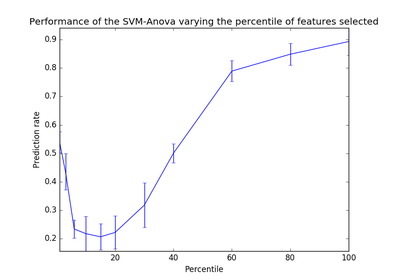SVM-Anova: SVM with univariate feature selection